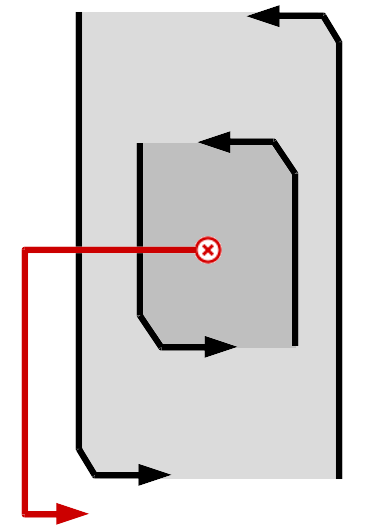# Programming-Idioms#### History of Idiom 43 > diff from v44 to v45

Edit summary for version 45 :
[Cpp] The comment provides an unrelated opinion that has nothing to do with the solution.

#### Version 44

2019-09-26, 17:31:27

#### Version 45

2019-09-26, 17:38:12

# Idiom #43 Break outer loop

Look for a negative value v in 2D integer matrix m. Print it and stop searching.# Idiom #43 Break outer loop

Look for a negative value v in 2D integer matrix m. Print it and stop searching.##### Imports
`#include <iostream>`
##### Imports
`#include <iostream>`
##### Code
```auto indices = findNegativeValue (m, 10, 20);
std::cout << m[indices.first][indices.second] << '\n';

std::pair<int, int> findNegativeValue (int **m, int rows, int columns) {
for (int i = 0; i < rows; ++i) {
for (int j = 0; j < columns; ++j) {
if (m[i][j] < 0) return make_pair (i, j);
}
}
throw "No negative value!";
}```
##### Code
```auto indices = findNegativeValue (m, 10, 20);
std::cout << m[indices.first][indices.second] << '\n';

std::pair<int, int> findNegativeValue (int **m, int rows, int columns) {
for (int i = 0; i < rows; ++i) {
for (int j = 0; j < columns; ++j) {
if (m[i][j] < 0) return make_pair (i, j);
}
}
throw "No negative value!";
}```